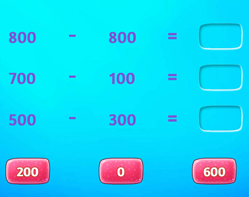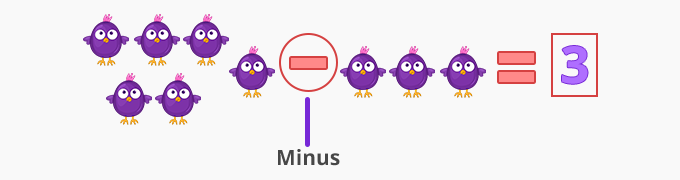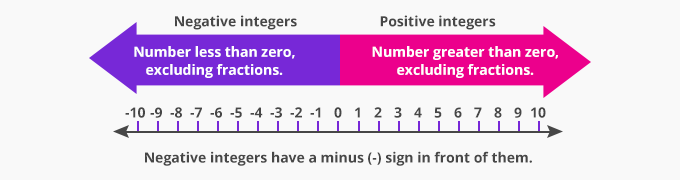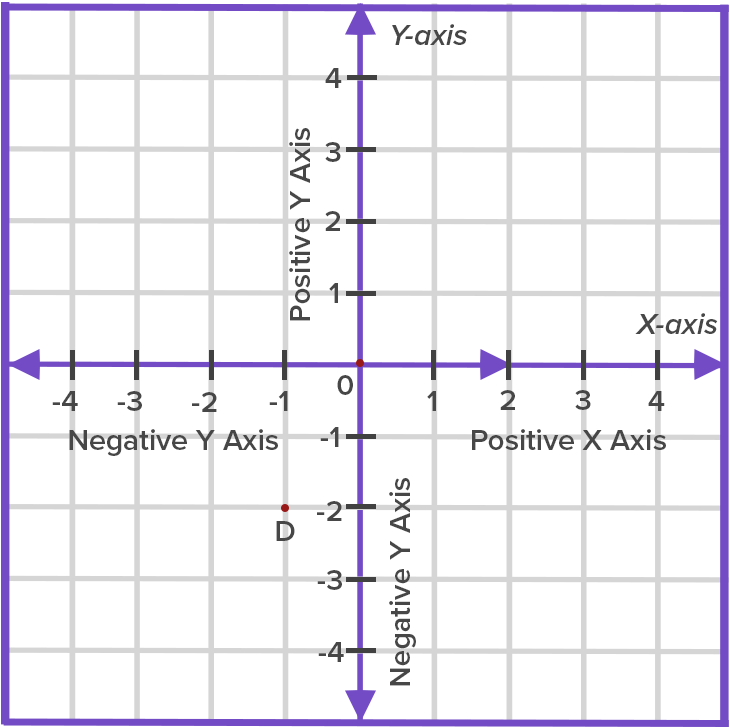# Minus - Definition with Examples

The Complete K-5 Math Learning Program Built for Your Child

• 40 Million Kids

Loved by kids and parent worldwide

• 50,000 Schools

Trusted by teachers across schools

• Comprehensive Curriculum

Aligned to Common Core

## Minus GamesSubtract Multiples of 100

Practice subtracting a multiple of 100 from another. For example, 300 - 200 = 100 can be thought of as 3 hundreds - 2 hundreds = 1 hundred.

Covers Common Core Curriculum 2.NBT.7

## What is Minus?

Minus sign is used in mathematics for multiple representations.

• Subtraction operation

Minus represents the arithmetic operation of subtraction between two numbers. For example,Minus sign also means taking something away from a given value.

For example, Darby has 6 gingerbread with her and she gives 3 gingerbread to Kelly.

So 6 minus 3 is equal to 3. Darby is left with 3 gingerbread.• To represent negative integers

The minus sign is used to represent negative integers, whole numbers less than zero (no fractions).For example, negative integer 3 is represented as: -3

• Mathematical operations on integers using ‘Minus’ sign

Multiplication of two negative numbers gives a positive.

Minus x Minus  = Positive

For example,

- 4 -10 = + 40

• Use in measurement

For example, A temperature of  - 3˚ C means 3 degrees below zero.

Another example,

The temperature is 4˚ C and then drops -10˚. What is the temperature now?

4 - 10 = -6• To represent opposite directions

We use the minus sign to represent a negative direction on a graph paper.It also runs in the negative direction.

Won Numerous Awards & Honors